Name:    Practice Test 3B

Multiple Choice

1.

Zinc oxide contains 80.3% zinc by mass.  What size sample of zinc oxide would contain 5.35 g of zinc?
 A) 27.2 g B) 4.30 g C) 6.66 g D) 11.0 g

2.

Which statement is correct about the reaction shown below:

2 Na (s)  +  Cl2 (g)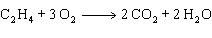2 NaCl (s)
 A) Sodium is reducted and chlorine is oxidized. B) Sodium is oxidized and chlorine is reduced C) Sodiuim and chlorine are both oxidized D) Sodium and chlorine are both reduced.

3.

How many grams of oxygen are needed to completely react with 200.0 g of NH3, as shown below?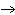A) 3.406 g B) 300.6 g C) 469.7 g D) 2.180 g

4.

In the reaction below, what substance is reduced?

SiO2 (s)  +  2 C (s)Si (s) + 2 CO (g)
 A) Si B) C C) O D) CO

5.

What is the correct classification for the reaction shown below?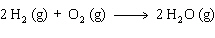A) decomposition B) double replacement C) single replacement D) synthesis

6.

Which of the following is the correctly balanced equation for decomposition of potassium chlorate (KClO3) to form potassium chloride and oxygen?
 A) KClO3 (s)KCl (s) + 3 O (g) B) KClO3 (s)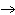KCl (s) + O3 (g) C) 2 KClO3 (s)K2Cl2 (s) + 3 O2 (g) D) 2 KClO3 (s)2 KCl (s) + 3 O2 (g)

7.

Which of the following equations is a correct oxidation half-reaction?
 A)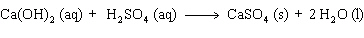B)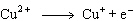C)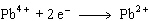D)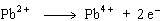8.

Which of the following reactions will NOT occur?
 A) I2 + 2 HCl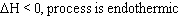2 HI + Cl2 B) Zn + PbCl2 ®  ZnCl2 + Pb C) Mg + ZnSO4Zn  + MgSO4 D) Pb + 2 HCl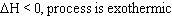PbCl2 + H2

9.

What mass of Mg is present in 25.5 g of magnesium chloride (MgCl2)?
 A) 6.51 g B) 24.3 g C) 8.94 g D) 15.5 g

10.

Cold packs, used in athletic and medical applications, work by lowering temperature when ammonium nitrate is dissolved in water.  Which of the following statements is true for this process?
 A)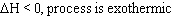B)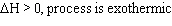C)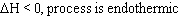D)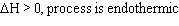11.

How many moles of water are produced when 2.50 moles of oxygen react with excess hydrogen as shown below: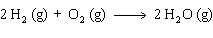A) 2.00 mol B) 1.25 mol C) 5.00 mol D) 3.50 mol

12.

Which of the following statements is correct?
 A) Adding Pb to ZnSO4 will produce Zn B) Adding Cu to HCl will release hydrogen gas C) Adding Fe to MgBr2 will produce Mg D) Adding Cu to AgC2H3O2 will produce silver

13.

How many moles of CO2 can be produced by the reaction of 5.00 mol of C2H4 and 12.0 mol of O2 as shown below?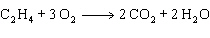A) 4.00 mol B) 5.00 mol C) 8.00 mol D) 10.0 mol

14.

The thermite reaction, shown below, is highly exothermic and used by railroad repair crews to wels rails together.  What mass of iron is formed when 725 kJ of heat are released?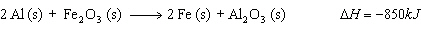A) 130 g B) 95 g C) 65 g D) 47 g

15.

How many moles of NH3 are needed to react completely with 13.6 mol of F2, as shown below?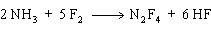A) 6.80 mol B) 2.27 mol C) 34.0 mol D) 5.44 mol